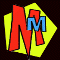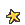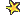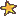The MISSING DIGIT trick!If you've read NUMBERS - the Key to the Universe you'll already know what a strange number 9 is. There are lots of tricks you can do with it, but when the mm author Kjartan was visiting South Leeds High School, he was shown a fast version of one of the tricks by Sadikul Haque.Here it is!

• Go and hide in a cupboard, or cover your eyes in some way so that you can't see what your friend is writing.
• Ask a friend to secretly write down ANY number (at least four digits long). e.g. 78341
• Ask the friend to add up the digits... e.g. 7+8+3+4+1 = 23
• ... and then subtract the answer from the first number.e.g. 78341 - 23 = 78318
• Your friend then crosses out ONE digit from the answer. (It can be any digit except a zero) e.g. 7x318
• Even though you haven't seen any numbers, you can say what the missing digit is! EIGHTTHE SECRET

This great trick relies on the power of 9.

• After your friend has added up the digits and subtracted them, the answer will ALWAYS divide by 9. If a number divides by nine, then when you add the digits up, they will also divide by 9. If you check our example 7+8+3+1+8 = 27 which does divide by nine.
• When your friend crosses a digit out, he then reads out the digits that are left. You add them up. In the example we had 7+3+1+8 = 19
• All you do now is see what you have to add on to your answer to get the next number that divides by nine! The next number to divide by 9 after 19 is 27. So you need to add on EIGHT. This is the number that was crossed out!

Here's another example:
Say the number written down is 873946284 (urk!).Many thanks to Sadikul for showing us this, and also to his Dad for showing him!